How Cheenta works to ensure student success?
Explore the Back-Story

# NMTC 2017 Stage II - BHASKARA (Class 9, 10) - Problems and Solutions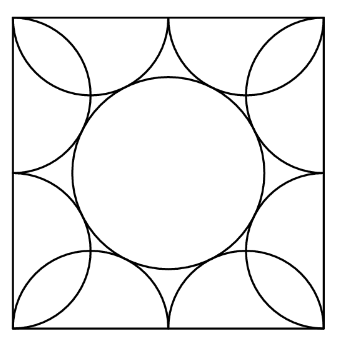###### Problem 1

(a) Find all prime numbers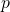such that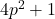andare also primes.
(b) Determine real numbers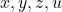such that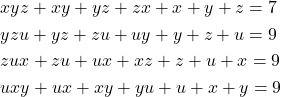###### Problem 2

If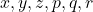are distinct real numbers such that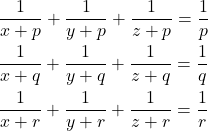find the numerical value of.

###### Problem 3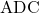and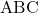are triangles such that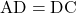and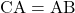. If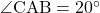and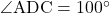, without using Trigonometry, prove that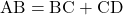.

###### Problem 4

(a) a, b, c, d are positive real numbers such that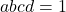. Prove that(b) In a scalene triangle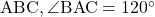. The bisectors of the angles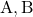and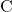meet the opposite sides in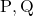and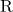respectively. Prove that the circle on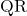as diameter passes through the point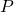.

###### Problem 5

(a) Prove thatcannot be expressed as a product of two polynomials of degree 2 with integer coefficients.
(b)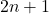segments are marked on a line. Each of these segments intersects at least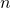other segments. Prove that one of these segments intersects all other segments.

###### Problem 6

If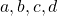are positive real numbers such that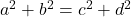and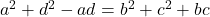, find the value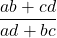###### Problem 1

(a) Find all prime numberssuch thatandare also primes.
(b) Determine real numberssuch that###### Problem 2

Ifare distinct real numbers such thatfind the numerical value of.

###### Problem 3andare triangles such thatand. Ifand, without using Trigonometry, prove that.

###### Problem 4

(a) a, b, c, d are positive real numbers such that. Prove that(b) In a scalene triangle. The bisectors of the anglesandmeet the opposite sides inandrespectively. Prove that the circle onas diameter passes through the point.

###### Problem 5

(a) Prove thatcannot be expressed as a product of two polynomials of degree 2 with integer coefficients.
(b)segments are marked on a line. Each of these segments intersects at leastother segments. Prove that one of these segments intersects all other segments.

###### Problem 6

Ifare positive real numbers such thatand, find the valueThis site uses Akismet to reduce spam. Learn how your comment data is processed.

### Knowledge Partner